Volume 4 Supplement 1

# Strategy and model building in the fourth dimension: a null model for genotype × age interaction as a Gaussian stationary stochastic process

## Abstract

### Background

Using univariate and multivariate variance components linkage analysis methods, we studied possible genotype × age interaction in cardiovascular phenotypes related to the aging process from the Framingham Heart Study.

### Results

We found evidence for genotype × age interaction for fasting glucose and systolic blood pressure.

### Conclusions

There is polygenic genotype × age interaction for fasting glucose and systolic blood pressure and quantitative trait locus × age interaction for a linkage signal for systolic blood pressure phenotypes located on chromosome 17 at 67 cM.

## Background

The Framingham Heart Study (FHS)  offers a unique opportunity to assess possible genotype × age (G × age) interaction in the genetic architecture of complex traits. To study G × age interaction in the FHS, we built on the variance components model , which, assuming negligible dominance, may be given as: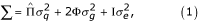where Σ is the variance-covariance matrix of a pedigree,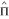is a matrix of estimated elements,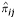, giving the proportion of alleles identical by descent (IBD) in individuals i and j at a quantitative trait locus (QTL), Φ is the kinship matrix of the pedigree, I is the identity matrix, and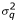,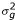, and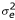are variances of the additive QTL, polygenic and environmental factors, respectively.

Our study was carried out in two phases. In Phase 1, we made inferences on polygenic G × age interaction using what we call the G × age model . Also, we experiment with correlation functions in the G × age model. In Phase 2, we implement the QTL version of this model. Multivariate variance components linkage analysis  can also be used to detect G × age interaction. We show how the bivariate variance components model can be used to detect QTL × age interaction. Given that the G × age and bivariate models apply to cross-sectional and longitudinal data, respectively, we compare their relative abilities to detect G × age interaction.

## Models and Methods

Using the bivariate mixed model , Blangero  derived the following expression for the genetic variance in phenotype response to two different environments: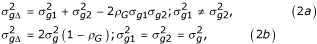where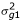and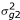are the additive genetic variances of the trait in Environments 1 and 2, in this case two ages,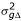is the additive genetic variance of the phenotypic difference between environments (i.e., trait response), and ρG is the genetic correlation between environments, in this case ages. The term environment, denoted by E, is used in accordance with standard statistical genetic theory . There is no G × E interaction (i.e.,= 0) if==and ρG = 1 . To model= 0,and ρG are parameterized as functions of age, which is the environment of interest in our analyses: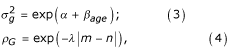whereis parameterized as an exponential function  and ρG as exponential decay , and α, β, and λ are parameters to be estimated, and m and n are two ages. Thus, the null hypotheses are β = 0 and λ = 0, which reflect a stationary covariance function.

In our analyses, we used the expected covariance matrix for a given pedigree , which has elements giving the covariance in trait value y for any two relatives:where x and z index individuals at ages m and n, respectively, Φ xz gives their kinship status, π is the probability that a random allele is IBD at the QTL, δxz is 1 for x = z and 0 otherwise, subscripts g, q, and e denote the polygenic, QTL, and environmental components, respectively, and average age is taken over the sample population, defined as all individuals measured for the trait of interest. Some points of clarification are needed. This is a cross-sectional model that applies generally to three types of pair-wise comparisons of individuals. In one type, let x = z such that m = n. Equation (5) gives the variances in this situation, in accord with the standard variance components model. In a second type, it may be such that x ≠ z while m ≠ n, and, in a third type, it may be such that x ≠ z while m ≠ n. Note that none of these types are longitudinal comparisons, which would be the case where x = z while m ≠ n (i.e., the same individual is measured at different ages). The goal of this approach lies in estimation of the change parameters, namely β and λ, so that we can test the null hypotheses stated above.

For the bivariate model, a trait measured at two different time points is treated as a bivariate phenotype. The pedigree covariance matrix model for a bivariate phenotype K, with constituent phenotypes I and J, may be written as :

Σ K = 2Φ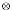G +Q + IE,     (6)

where the new matrices G, Q, and E convey the polygenic, QTL, and environmental variance components, respectively, andis the Kronecker-product operator. The variance components for this model are those for the univariate model for traits I and J, given by ΣI and ΣJ (cf. equation (1)), and the trait cross-covariances, which may be parameterized as ρgijσgiσgj, ρqijσqiσqj, and ρeijσeiσej for the polygenic, QTL, and environmental components, respectively . The null hypotheses of no polygenic genotype or QTL × age interaction are expressed as ρgij = 1 and ρqij = 1, respectively.

Scatter plots were examined for traits showing increasing or decreasing variance in trait values with age, suggesting potential for G × age interaction. Traits meeting these criteria, namely systolic blood pressure (SBP) and fasting glucose (GLUC), were analyzed using SOLAR , with age, sex, hypertension medication, and body mass index (BMI) as covariates. Phase 1 analyses were conducted on an augmented data set. Exams 12 (1970), 16 (1978), 18 (1982), and 20 (1986) in Cohort 1 were combined with Exams 1 (1971), 2 (1979), 3 (1983), and 4 (1987) in Cohort 2, respectively. To reduce kurtosis after combining, a few outliers were removed for SBP (1–6 per exam, all with values > the mean) but many for GLUC (51–101 per exam, all but one > the mean). No data transformation was made. Since diabetic status was not available, we could not control for it. This gave combined measurement periods 1–4.

Based on genome scans that we conducted for both SBP and GLUC, we decided to focus on SBP. For Phase 2, data for SBP values corrected for hypertension treatment for Cohorts 1 and 2, imputed following Levy et al.  (see Soler and Blangero ), were analyzed. Bivariate analyses were performed on residuals from multiple regressions with corrected SBP values as the dependent variable and age, sex and BMI as independent variables. For the bivariate analyses, analysis of residuals was necessary due to the unbalanced and incomplete structure of the FHS longitudinal data, which made covariate specification unrepresentative across measurements. For this SBP data set (corrected values and residuals), we experimented with combined exams from Cohorts 1 and 2 and with exams from Cohort 2 taken separately.

## Results

### Phase 1

We implemented equation (5) using three different correlation functions, specifically the standard normal, Cauchy, and exponential. These gave consistent results (not shown). Only selected results for the exponential function given by equation (4) are reported. For SBP, only combined measurement period 3 gave strong evidence for polygenic G × age interaction (Fig. 1). For GLUC, combined measurement periods 2–4 all gave strong evidence for polygenic G × age interaction and we believe combined measurement period 3 to be representative (Fig. 1).

The p-values reported in Figure 1 and Tables 1,2 are for the likelihood ratio statistic: Λ = -2 [ln L(θN)- ln L(θA)], where the null, HN (parameter constrained to 0 or 1 as appropriate), is compared to the alternative, HA (parameter estimated). In general, Λ is distributed as a χ2 random variable with degrees of freedom (d.f.) equal to the difference in the number of parameters under the null and alternative hypotheses . If parameter values are constrained to a boundary under the null hypothesis, the asymptotic distribution of Λ is given by a mixture of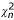random variables, where n denotes the d.f. and where the mixture may include n = 0 (point mass at 0) . However, the traditional criterion (i.e., difference in parameters) is conservative .

### Phase 2

We recovered the linkage signal for SBP on chromosome 17 at 67 cM reported by the FHS group  (Fig. 2; all maximum LOD scores > 3). We then applied the G × age and bivariate models in search of QTL × age interaction. Table 1 contains model selection criteria  and selected likelihood ratio tests for univariate analyses of corrected SBP values using the G × age model for combined Cohorts 1 and 2, Exams 20 and 4, respectively. Table 2 contains similar information for bivariate analyses of corrected SBP residuals using the bivariate model for Cohort 2, Exams 4 and 5.

## Conclusion

Our results suggest that there is polygenic G × age interaction for both GLUC and SBP (Fig. 1; Tables 1,2) and QTL × age interaction for the putative QTL for SBP phenotypes on chromosome 17 at 67 cM (linkage: Fig. 2; interaction: Table 1). To our knowledge, this is the first demonstration of QTL × age interaction in humans using linkage analysis methods.

The cross-sectional and longitudinal analyses do not give the same results. The difference may mean that cross-sectional data are better than longitudinal data at capturing G × age interaction. However, based on the received wisdom regarding the relative utility of cross-sectional and longitudinal analyses , this explanation does not seem tenable. Alternatively, that the bivariate model did not detect QTL × age interaction (Table 2) may simply be due to the loss in power with an overly parameterized model . Another explanation is that the bivariate model is really operating on time rather than on age and so perhaps the brief time span between Cohort 2, Exams 4 and 5, was too short to capture an interaction effect. Yet another explanation of the difference between the cross-sectional and longitudinal analyses is that the latter was carried out on Cohort 2 only, which, taken by itself, yielded a smaller sample size. Lastly, there is the possibility of some mixture of the last three problems.

Given that equations (5) and (6) derive from the same underlying modeling framework – namely variance components – they can be conceptualized as a strategy for testing the null hypothesis that a given phenotype "translated along the time axis" is a Gaussian covariance stationary stochastic process . This is now an established approach in statistical genetics , and is readily extended to the genetics of aging. It is our hope that the analyses herein contribute to a better understanding of the genetic architecture of the complex traits associated with the aging process and associated complex diseases (e.g., cardiovascular disease). We conclude that the G × age and bivariate models offer a feasible system for model building in the fourth dimension.

## References

1. Cupples LA, Yang Q, Demissie S, Copenhafer D, Levy D, for the Framingham Heart Study Investigators: Description of the Framingham Heart Study Data for Genetics Analysis Workshop 13. BMC Genetics. 2003, 4 (suppl 1): S2-10.1186/1471-2156-4-S1-S2.

2. Almasy L, Blangero J: Multipoint quantitative-trait linkage analysisin general pedigrees. Am J Hum Genet. 1998, 62: 1198-1211. 10.1086/301844.

3. Almasy L, Towne B, Peterson C, Blangero J: Detecting genotype × age interaction. Genet Epidemiol. 2001, 21 (suppl 1): S819-S824.

4. Almasy L, Dyer TD, Blangero J: Bivariate quantitative trait linkage analysis: pleiotropy versus co-incident linkages. Genet Epidemiol. 1997, b: 953-958. 10.1002/(SICI)1098-2272(1997)14:6<953::AID-GEPI65>3.0.CO;2-K.

5. Blangero J, Konigsberg LW: Multivariate segregation analysis using the mixed model. Genet Epidemiol. 1991, 8: 299-316. 10.1002/gepi.1370080503.

6. Blangero J: Statistical genetic approaches to human adaptability. Hum Biol. 1993, 65: 941-966.

7. Davidian M, Carroll RJ: Variance function estimation. J Am Statist Assoc. 1987, 82: 1079-1091. 10.2307/2289384.

8. Lange K: Cohabitation, convergence, and environmental covariances. Am J Med Genet. 1986, 24: 483-491. 10.1002/ajmg.1320240311.

9. Levy D, DeStefano AL, Larson MG, O'Donnell CJ, Lifton RP, Gavras H, Cupples LA, Myers RH: Evidence for a gene influencing blood pressure on chromosome 17: genome scan linkage results for longitudinal blood pressure phenotypes in subjects from the Framingham Heart Study. Hypertension. 2000, 36: 477-483.

10. Soler JMP, Blangero J: Longitudinal familial analysis of blood pressure involving parametric (co)variance functions. BMC Genetics. 2003, 4 (suppl 1): S87-10.1186/1471-2156-4-S1-S87.

11. Self SG, Liang K-Y: Asymptotic properties of maximum likelihood estimators and likelihood ratio tests under nonstandard conditions. J Am Statist Assoc. 1987, 82: 605-610. 10.2307/2289471.

12. Burnham KP, Anderson DR: Model Selection and Multimodel Inference: A Practical Information-Theoretic Approach. New York, Springer-Verlag. 2002, 2

13. Ware JH: Linear models for the analysis of longitudinal studies. Am Statist. 1985, 39: 95-101. 10.2307/2682803.

14. Pletcher SD, Geyer CJ: The genetic analysis of age-dependent traits: modeling the character process. Genetics. 1999, 151: 825-835.

## Acknowledgments

We thank Drs. Jean W. MacCluer and Ravindranath Duggirala for critically reviewing the manuscript. This work is supported in part by NIH R01MH59490.

## Author information

Authors

### Corresponding author

Correspondence to Vincent P Diego.

## Rights and permissions

Reprints and Permissions

Diego, V.P., Almasy, L., Dyer, T.D. et al. Strategy and model building in the fourth dimension: a null model for genotype × age interaction as a Gaussian stationary stochastic process. BMC Genet 4 (Suppl 1), S34 (2003). https://doi.org/10.1186/1471-2156-4-S1-S34